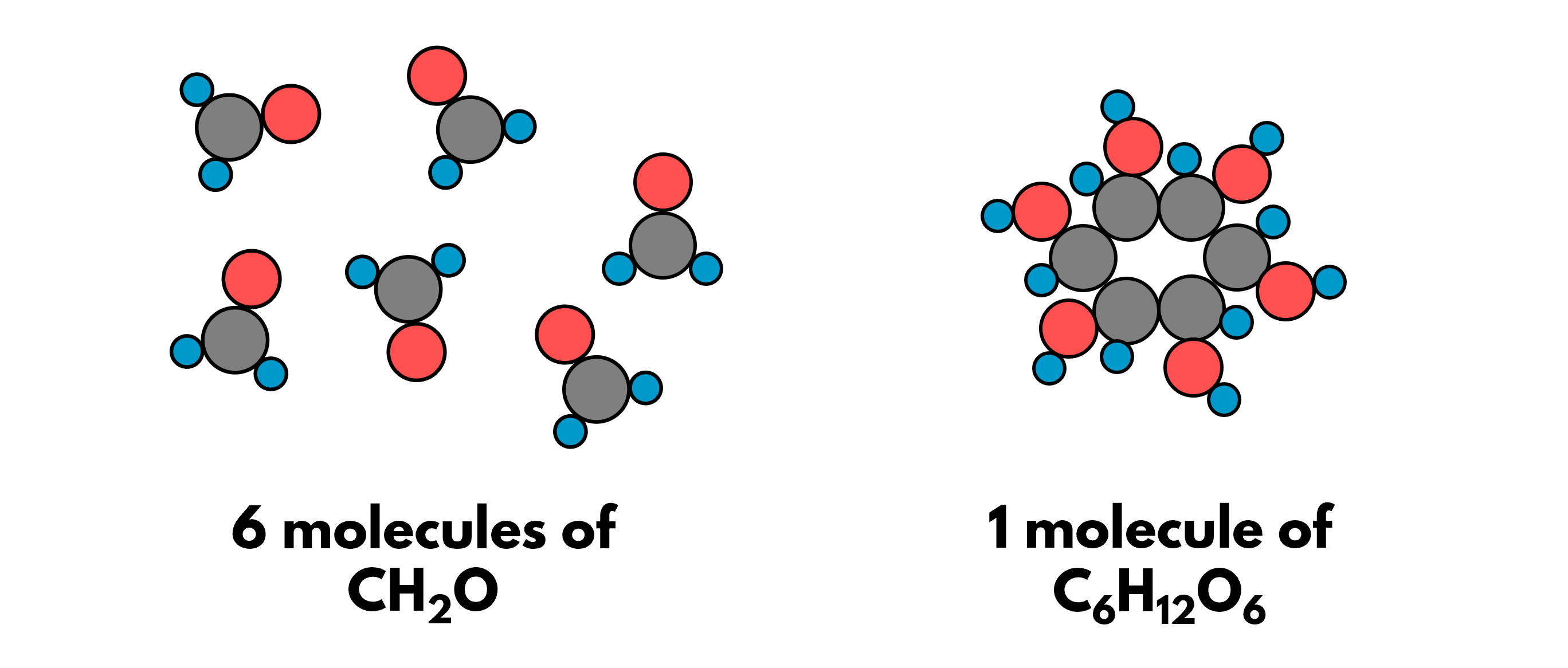## 1. The iconic duo

There cannot be a more iconic duo, in Chemistry at least, than the sodium ion and chloride ion in the compound sodium chloride.

The ions come in pairs. We know this from the chemical formula of NaCl, whereby the ratio of sodium ions to chloride ions is 1:1. Therefore, in terms of number of particles, 50% of the particles in the giant ionic lattice are sodium ions, while the remaining 50% are chloride ions.

## 2. Elements pulling their weight

There is another way to look at the composition of sodium chloride. Sodium, being the lighter element, takes up 39% of the total mass of the compound. Chlorine, the heavyweight, takes up the remaining 61% of the total mass. We call this the percentage mass of an element in a compound.

How did we get these percentages? We express the mass of all the atoms of the element we are interested in as a fraction of the total mass of the compound:

% mass of element Z = (no. of atoms of Z × Ar of Z) ÷ Mr of compound × 100%

## 3. Calculating percentage mass from chemical formula

Let’s practise by working with something complicated: to find the percentage mass of carbon in glucose molecule, C6H12O6. We shall break up the above method into three separate steps:

• ### STEP 1: Find the relative molecular mass, Mr of C6H12O6

Mr = 6×12 + 12×1 + 6×16 = 180

• ### STEP 2: Find the relative mass of all carbon atoms in a molecule of C6H12O6

Relative mass of carbon in C6H12O6 = 6×12 = 72

• ### STEP 3: Compute the percentage mass

% mass of C = (72÷180) × 100% = 40%

## 4. Working backwards in the laboratory to find empirical formula

If we can use the chemical formula of compounds to calculate the percentage mass of each of their constituent element, we can work backwards! In the laboratory, we can measure the percentage mass of each element to infer the chemical formula. We call this formula the empirical formula.

Yes, we heard you! What does that cheem word mean? Empirical is the opposite of theoretical. It means that the formula is our intelligent guess based on first-hand observations made in the laboratory.

To infer the empirical formula, we shall:

1. Assume that you are working with a 100 g sample, express percentage mass as mass
2. Convert mass into number of moles: mass ÷ molar mass = number of moles
3. Divide the large number of moles by the smallest number of moles
4. Find the simplest mole ratio in whole numbers

## 5. I spy empirically with my little eye…

Let’s say we found out from experiments that an unknown sugar has 40% carbon, 6.7% hydrogen, and 53.3% oxygen. From this, we can infer the empirical formula by following the above steps:

Therefore, the empirical formula of the unknown sugar is CH2O.

## 6. Big brain time: finding molecular formula from empirical formulaTwo very different compounds – CH2O and C6H12O6 – have the same percentage mass of each element and hence the same empirical formula

Empirical formula is the simplest ratio of elements. It may not show the actual number atoms in one molecule of the compound. In other words, for the empirical formula of CH2O that we found, the actual molecular formula may be: CH2O, C2H4O2, C3H6O3, C4H8O4, C5H10O5, C6H12O6CnH2nOn. In other words, the molecular formula is one of the multiples of the simplest ratio of 1:2:1. We shall represent the multiple as an unknown variable, n.

To correctly identify the molecular formula is to find the value of n. We will need another piece of experimental result to do so: the relative molecular mass, Mr.

n × relative mass of atoms in empirical formula = actual Mr of compound

Given that the Mr of the unknown sugar is 180:

• ### STEP 1: Find the relative mass of atoms in the empirical formula of CH2O

Relative mass of atoms = 12 + 2×1 + 16 = 30

• ### STEP 2: Given that the molecular formula is CnH2nOn, find the value of the multiplier, n

n × 30 = 180
n = 180 ÷ 30 = 6

C6H12O6